# Cyclic Code Linear Block Code Hamming Code is

• Slides: 21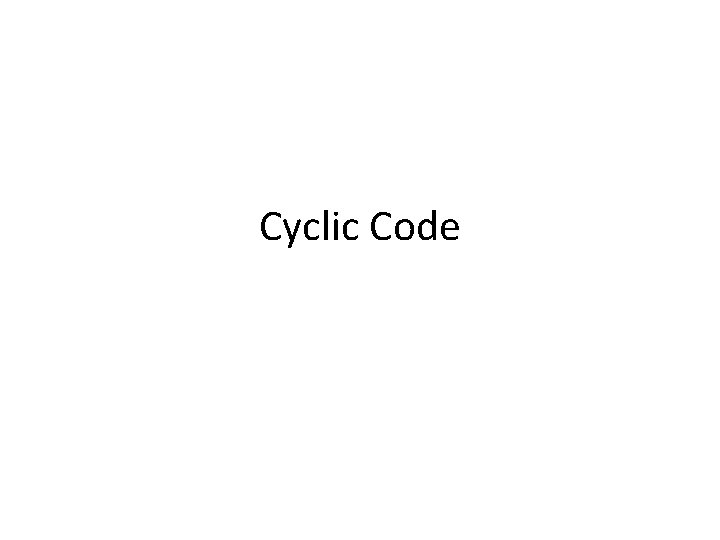Cyclic CodeLinear Block Code • Hamming Code is a Linear Block Code means that the codeword is generated by multiplying the message vector with the generator matrix. • Minimum weight as large as possible. If minimum weight is 2 t+1, capable of detecting 2 t error bits and correcting t error bits.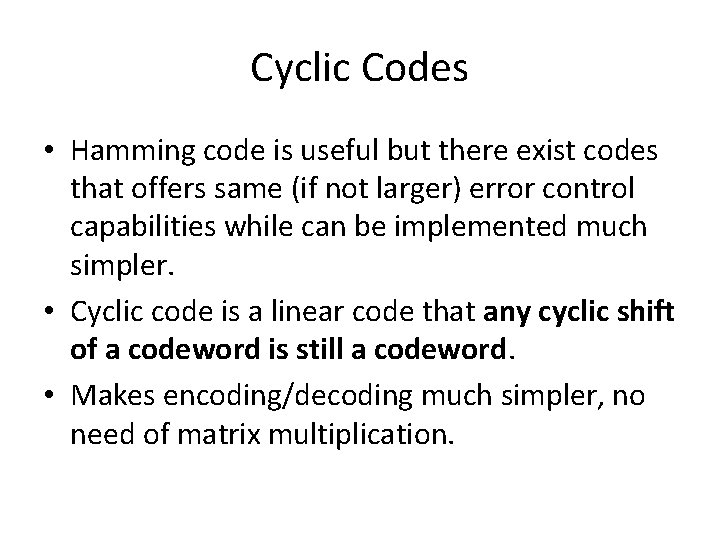Cyclic Codes • Hamming code is useful but there exist codes that offers same (if not larger) error control capabilities while can be implemented much simpler. • Cyclic code is a linear code that any cyclic shift of a codeword is still a codeword. • Makes encoding/decoding much simpler, no need of matrix multiplication.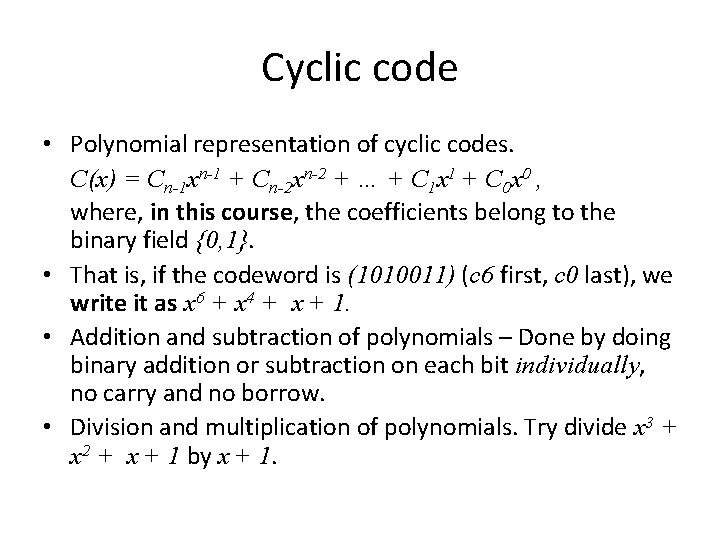Cyclic code • Polynomial representation of cyclic codes. C(x) = Cn-1 xn-1 + Cn-2 xn-2 + … + C 1 x 1 + C 0 x 0 , where, in this course, the coefficients belong to the binary field {0, 1}. • That is, if the codeword is (1010011) (c 6 first, c 0 last), we write it as x 6 + x 4 + x + 1. • Addition and subtraction of polynomials – Done by doing binary addition or subtraction on each bit individually, no carry and no borrow. • Division and multiplication of polynomials. Try divide x 3 + x 2 + x + 1 by x + 1.Cyclic Code • A (n, k) cyclic code can be generated by a polynomial g(x) which has – degree n-k and – is a factor of xn - 1. Call it the generator polynomial. • Given message bits, (mk-1…m 1 m 0 ), the code is generated simply as: • In other words, C(x) can be considered as the product of m(x) and g(x).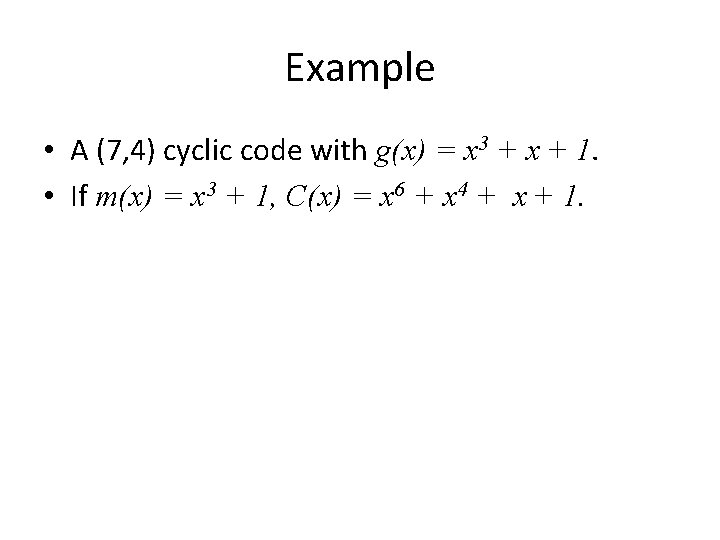Example • A (7, 4) cyclic code with g(x) = x 3 + x + 1. • If m(x) = x 3 + 1, C(x) = x 6 + x 4 + x + 1.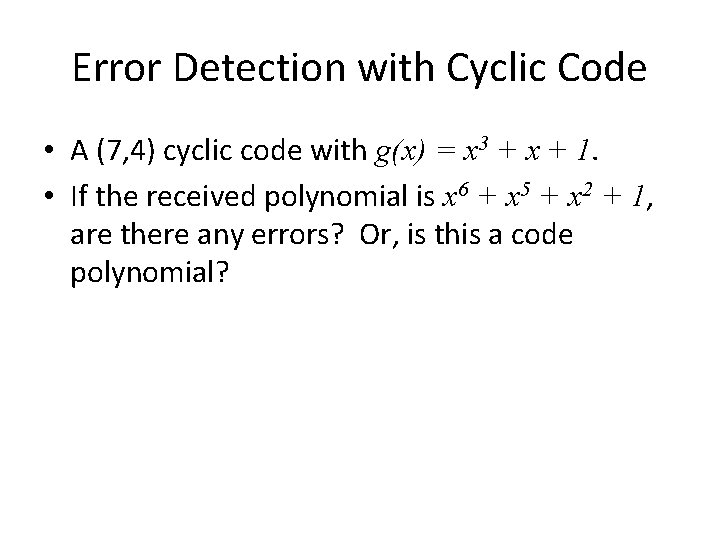Error Detection with Cyclic Code • A (7, 4) cyclic code with g(x) = x 3 + x + 1. • If the received polynomial is x 6 + x 5 + x 2 + 1, are there any errors? Or, is this a code polynomial?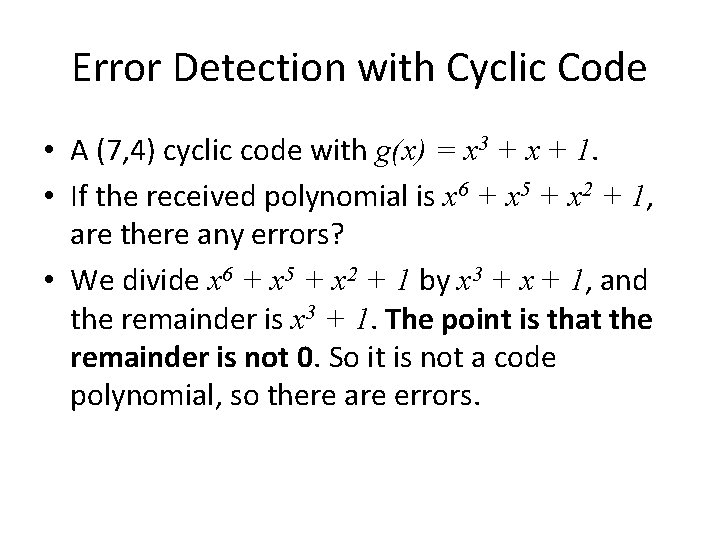Error Detection with Cyclic Code • A (7, 4) cyclic code with g(x) = x 3 + x + 1. • If the received polynomial is x 6 + x 5 + x 2 + 1, are there any errors? • We divide x 6 + x 5 + x 2 + 1 by x 3 + x + 1, and the remainder is x 3 + 1. The point is that the remainder is not 0. So it is not a code polynomial, so there are errors.Cyclic code used in IEEE 802 • g(x) = x 32 + x 26 + x 23 + x 22 + x 16 + x 12 + x 11 + x 10 + x 8 + x 7 + x 5 + x 4 + x 2 + x + 1 – all single and double bit errors – all errors with an odd number of bits – all burst errors of length 32 or less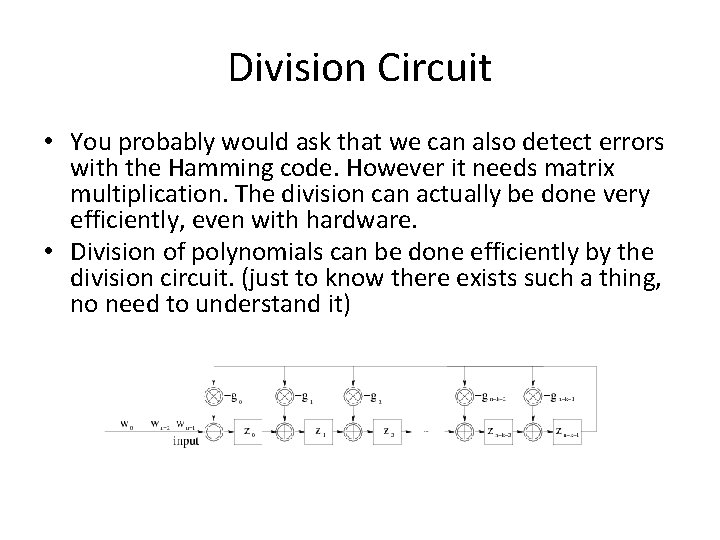Division Circuit • You probably would ask that we can also detect errors with the Hamming code. However it needs matrix multiplication. The division can actually be done very efficiently, even with hardware. • Division of polynomials can be done efficiently by the division circuit. (just to know there exists such a thing, no need to understand it)Cyclic Code • One way of thinking it is to write it out as the generator matrix • So, clearly, it is a linear code. Each row of the generator matrix is just a shifted version of the first row. Unlike Hamming Code. • Why is it a cyclic code?Example • The cyclic shift of C(x) = x 6 + x 4 + x + 1 is C 1(x) = x 5 + x 2 + x + 1. • It is still a code polynomial, because the code polynomial is m(x) = x 2 +1.Cyclic Code • Given a code polynomial • We have • Therefore, C 1(x) is the cyclic shift of C(x) and • has a degree of no more than n-1 • divides g(x) (why? ) hence is a code polynomial.Cyclic Code • To generate a cyclic code is to find a polynomial that – has degree n-k – is a factor of xn -1.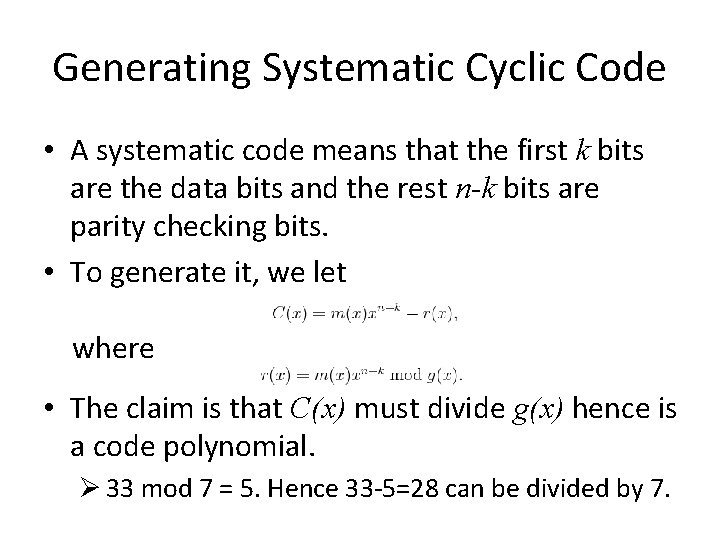Generating Systematic Cyclic Code • A systematic code means that the first k bits are the data bits and the rest n-k bits are parity checking bits. • To generate it, we let where • The claim is that C(x) must divide g(x) hence is a code polynomial. Ø 33 mod 7 = 5. Hence 33 -5=28 can be divided by 7.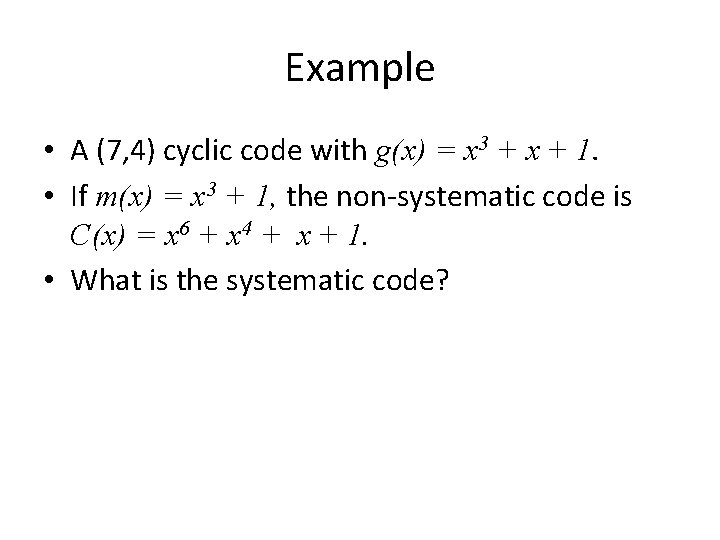Example • A (7, 4) cyclic code with g(x) = x 3 + x + 1. • If m(x) = x 3 + 1, the non-systematic code is C(x) = x 6 + x 4 + x + 1. • What is the systematic code?Example • A (7, 4) cyclic code with g(x) = x 3 + x + 1. • If m(x) = x 3 + 1, the non-systematic code is C(x) = x 6 + x 4 + x + 1. • What is the systematic code? • r(x) = m(x) x 3 mod g(x) = (x 6 + x 3) mod x 3 + x + 1 = x 2 + x • Therefore, C(x) = x 6 + x 3 + x 2 + x.Cyclic Redundancy Check (CRC) • In communications, usually the data is followed by a checksum. • Checksum is calculated according to a cyclic code, therefore it is called Cyclic Redundancy Check (CRC). • To be more precise, it is done by calculating the systematic code, with the data packet as the message polynomial. • The receiver, once received the data followed by the checksum, will calculate the checksum again, if match, assume no error, otherwise there is error, either in the data or the checksum.Research Challenge • In wireless communications, a packet of 1500 bytes usually has less then 10 byte errors, usually clustered in a few locations, if corrupted. • Standards today say retransmit everything. • Any better ideas?Remaining Questions for Those Really Interested • Decoding. Divide the received polynomial by g(x). If there is no error you should get a 0 (why? ). Make sure that the error polynomial you have in mind does not divide g(x). • How to make sure to choose a good g(x) to make the minimum degree larger? Turns out to learn this you have to study more – it’s the BCH code.Other codes • RS code. Block code. Used in CD, DVD, HDTV transmission. • LDPC code. Also block code. Reinvented after first proposed 40 some years ago. Proposed to be used in 802. 11 n. Achieve close-to-Shannon bound • Trellis code. Not block code. More closely coupled with modulation. • Turbo code. Achieve close-to-Shannon bound.